# HBSE 9th Class Science Important Questions Chapter 12 Sound

Haryana State Board HBSE 9th Class Science Important Questions Chapter 12 Sound Important Questions and Answers.

## Haryana Board 9th Class Science Important Questions Chapter 12 Sound

Question 1.
What is sound?
Sound is a kind of energy that is produced by vibration.

Question 2.
What is the state of the body that is producing sound?
Vibrating state.

Question 3.
How does sound travel in air?
Sound travels in compressions and rarefactions.

Question 4.
In which the speed of sound is more-solids, liquids or gases ?
In solids.

Question 5.
What is the speed of sound in air at 0°C ?
331 m/s.

Question 6.
What is the speed of sound in air at 22°C ?
344 m/s.Question 7.
What is the speed of sound in distilled water at 25°C ?
1498 m/s.

Question 8.
In which the speed of sound is 5100 m/s at 20°C ?
(i) steel
(ii) aluminium
(iii) alcohol
(iv) mercury.
(ii) aluminium.

Question 9.
What is the velocity of light ?
3 x 108 m/s.

Question 10.
Name two forms of energy.
(i) Heat energy
(ii) Light energy.

Question 11.
What is medium ?
The liquid or material through which sound propagates, is called medium.

Question 12.
What moves forward in sound propagation-particle of medium or disturbance ?
Disturbance moves forward.

Question 13.
Why are sound waves called mechanical waves ?
Because sound waves are characterise by the particles of medium. Therefore, the sound waves are called mechanical waves.Question 14.
What is compression ?
The region of high pressure in medium is called compression (C).

Question 15.
What is rarefaction ?
The region of low pressure in medium is called rarefaction (R).

Question 16.
What is the meant of 256 written on tuning fork ?
It means its frequency is 256 Hz.

Question 17.
What are electromagnetic waves ?
Those waves which can travel without any medium are called electromagnetic waves.

Question 18.
Can the sound waves travel in vacuum ?
No, sound waves cannot travel in vacuum.

Question 19.
Which type of waves are used by astronauts to talk on moon ?
Ans:

Question 20.
Define wave velocity.
The distance covered by the wave per unit time is called wave velocity.

Question 21.
What is the relation between frequency and time period ?
Frequency is the reciprocal of time period. That is v = $$\frac {1}{T}$$ .

Question 22.
What is the relation among velocity of wave, wavelength and frequency ?
The velocity of wave is equal to the product of frequency and wavelength. That v = v X

Question 23.
How many type of waves can slinky produce ?
Slinky can produce three types of waves :
(1) Transverse waves.
(2) Longitudinal waves.
(3) Periodic waves.Question 24.
Which physical quantity is propagated by waves ?
Energy.

Question 25.
What is the SI unit of frequency ?
Hertz.

Question 26.
What is the SI unit of wavelength ?
Metre.

Question 27.
What is the SI unit of wave velocity ?
Metre/second (m/s)

Question 28.
Define pulse.
Waves of shorter duration is called pulse.

Question 29.
What is the effect on the particles of the medium when a wave moves ?
When a wave moves, the particles of the medium execute only pendulum motion, and not moves with sound.

Question 30.
What are longitudinal waves ?
When the individual particles of the medium move in a direction parallel to the direction of propagation of disturbance are called
longitudinal waves.

Question 31.
How many types are there of waves ?
There are three types of waves:
(i) Longitudinal waves
(ii) Transverse waves
(iii) Periodic waves.

Question 32.
Which are longitudinal waves among these ?
(i) waves on pond water
(ii) sound waves
(iii) light waves.
(ii) Sound waves.Question 33.
What are transverse waves ?
When the individual particles of the medium moves in a direction perpendicular to the direction of wave propagation, are called
transverse waves.

Question 34.
The propagation of sound is the example of which wave ?
Longitudinal wave.

Question 35.
What types of waves are produced on pond water ?
Transverse waves.

Question 36.
How do the longitudinal waves travel ?
The longitudinal waves travel in compressions and rarefactions.

Question 37.
What is a trough ?
The maximum displacement in negative direction on Y-axis in the graph of periodic waves is called a trough.

Question 38.
Define the pitch.
How the brain interprets the frequency of an emitted sound is called the pitch.

Question 39.
Define note.
A sound which is produced due to a mixture of several frequencies is called a note.

Question 40.
What are the causes of variations in sounds ?
The main cause of variations in sounds are loudness of sound, quality, pitch of sound and frequency.

Question 41.
What is the hearing range of our ears ?
20 Hz to 20,000 Hz.

Question 42.
Define ultrasonic sound.
Frequencies higher than 20 kHz are called ultrasonic sound or ultrasound.

Question 43.
What is the frequency of the waves produced during earthquake and valcano eruption ?
Frequencies less than 20 Hz.Question 44.
Write the name of two creatures which produce sounds of frequencies less than 20 Hz.
(i) Whale
(ii) Elephant.

Question 45.
Write the name of two creatures which produce ultrasonic sound.
(i) Bats
(ii) Dogs.

Question 46.
Which sound helps the bat to locate their way ?
Ultrasound.

Question 47.
What is reverberation ?
The repeated reflection that results in the persistence of sound is called reverberation.

Question 48.
What is meant by supersonic speed ?
When the speed of any object exceeds the speed of sound it is said to be travelling at supersonic speed.

Question 49.
Which types of aircraft produce shock waves ?
Supersonic aircraft.

Question 50.
What is the sound called produced by motion of supersonic aircrafts ?
Sonic boom.

Question 51.
What is meant by reflection of sound ?
When sound strikes on a surface, it changes its direction, it is known as reflection of sound.

Question 52.
When sound produces in deep wells, huge buildings and mountains, we experience a sound after some time besides mean sound, what is that ?
Echo.

Question 53.
On which principle the megaphone works ?
Megaphone works on the principle of reflection of sound.

Question 54.
What should be the minimum time to have echo between mean sound and echo ?
0.1 second or $$\frac {1}{10}$$ second.

Question 55.
How much minimum distance should be their to listen echo from a reflecting object ?
17.2 metres.

Question 56.
What will be the minimum distance covered by the sound to hear echo ?
34.4 metres.

Question 57.
Define sonar.
Sonar means Sound Navigation And Ranging. It is a device that uses ultrasonic waves to measure the distance, direction and speed of underwater objects.Question 58.
Where is SONAR used ?
It is used to determine the depth of the sea and to locate underwater hill, valleys, submarine, icebergs and sunken ships, etc.

Question 59.
Which type of waves are used in SONAR system ?
Ultrasonic waves.

Question 60.
Upto what frequency, the bat can produce ultrasonic waves ?
Upto 120 kHz.

Question 61.
By emitting which waves to the painful part of body, we feel very like rubing (malish) ?
Ultrasound wave.

Question 62.
Why is it beneficial to use ultrasound instead of X-rays to take the photograph of inner body ?
Because ultrasound does not affect tissues and cells.

Question 63.
What is ultrasonography ?
The technique which is used to examine the foetus during pregnancy to detect congenial defects and growth abnormalities is called ultrasonography.

Question 64.
Which type of waves are used to locate the eye tumor and to cure that ?
Ultrasonic waves.

Question 65.
Which characterstics of ultrasound is used to develop photographic film ?
Mixing of immiscible liquids by ultrasound.

Question 66.
Which type of waves are used in breadth therapy ?
Ultrasonic waves.Question 1.
Prove that sound is a form of energy.
When we speak for long time or loudly, we get tired. We spend our energy during talking. This energy produces vibration in throat. It proves that sound is a form of energy.

Question 2.
How is sound produced ? Justify with example.
It is compulsory to vibrate for the production of sound. For example; when we speak, the vocal cord of throat vibrate. When trumphets or guitar play, its wires vibrate. When bell is rung, there is also vibrations. The vibrations in bell can be felt by touching it by hand. In this way, the sound is produced by vibrations.

Question 3.
Define compression and rarefaction. Draw a graph to show the density of air molecules due to sound waves.
Compression: A region of high pressure is called compression.
Rarefaction: A region of low pressure is called rarefaction.
The graph between the density of air in unit interval and distance can be drawn.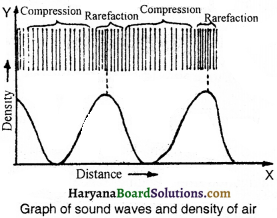In fig. the distribution of air atoms produced by sound propagation of sound wave is shown. The graph between density and distance is also shown.

Question 4.
Justify with an example that how the compression and rarefaction formed in vibrating body move ?
Experiment:
Take a spiral spring as shown in fig. Press one end of spring slowly by hand and then leave. We see that the compressions and rarefactions are formed in the spring and they propagate from one end to another end. The propagating motion of compressions and rarefactions is called wave motion. In the same way, the compressions and rarefactions produced in air propagate from one layer to another and in this way, the sound travels from one place to another.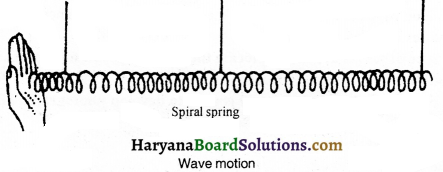Question 5.
Justify the sound system in air by tuning fork.
The prongs of tuning fork start vibrating when hit with a rubber pad. The vibrating prong exert pressure of air particles and displaces them. The air particles come in motion from rest and come in original postition after displacing the nearby particles. The compression of high pressure is formed at the region where the air particles come close, and after detaching pressure of vibration, the particles come in their original position. This region of low pressure is called rarefaction. The particles of medium do not move forward itself but disturbance of particle moves forward by compression and rarefaction. This is called sound wave. Air is the most common medium for the propagation of sound.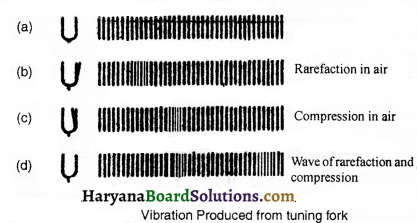Question 6.
Can sound make a light spot dance ? Justify it.
Take an empty tin with both ends open. Tie stretched membrane of balloon on one end. Take one piece of plane mirror and stick it to the membrane of balloon in such a way that its reflecting surface points upward and the rays coming from a source should fall on the mirror and after reflection the light spot should be on the wall. According to figure, make a loud sound from the open end of tin. You will see the light will dance.

Cause:
Due to vibration of sound, the membrane and the mirror on it vibrate, due to this the image formed on wall seems to be shaking. Stop the sound. The spot will be on fixed place because the vibration of membrane is stopped.Question 7.
Define longitudinal and transverse waves.
(i) Longitudinal wave : When the individual particles of the medium move in a direction parallel to the direction of propagation of the disturbance is called longitudinal wave.
(ii) Transverse wave : When the individual particles of the medium move in a direction perpendicular to the direction of wave propagation, is called transverse wave.

Question 8.
Define crest, trough and amplitude and show them for a transverse wave by graph.
The crest, trough and amplitude of a transverse wave are shown in fig.
Crest: Peaks formed during the motion of transverse wave is called crest.
Trough: The depression formed during the motion of transverse wave is called trough.
Amplitude: The maximum displacement of medium particles from their mean position during transmission of waves is called amplitude. The unit of amplitude is metre.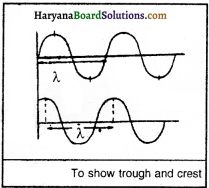Question 9.
Distinguish between longitudinal and transverse waves.
The differences between longitudinal and transverse waves are as follows

 Longitudinal waves Transverse waves 1. The individual particles of the medium move in a direction parallel to the direction of propagation of the disturbance in these waves. 1. The individual particles of the medium move in a direction perpendicular to the direction of propagation of the disturbance in these waves. 2. Compression and rarefaction are formed in it which make a wave. 2. Crest and trough are formed in it, which make a wave. 3. These waves can be formed in any medium, solid, liquid or gas. 3. Solid or liquid medium is necessary for the formation of these waves. 4. These waves cannot be polarised. 4. These waves can be polarised. Longitudinal waves: Transverse waves:

Question 10.
What do you know about these :
(i) Loudness of sound
(ii) Pitch
(iii) Frequency.
Or
Write two differences between the loudness and pitch of sound.
(i) Loudness of sound:
The level of the sensation of the sound is called the loudness of sound. This level of sensation depends upon the sharp or light vibrations of source. When vibrations are more, the loudness of sound will be more and when vibrations are light, the loudness of sound will be low.(ii) Pitch:
That property’of sound by which we can differentiate between the louder and softer sound. This property depends upon the frequency of the source of sound. When the frequency of the source of sound is more, the pitch is also more.

(iii) Frequency:
The number of vibrations completed by any object in one second is called frequency.

Question 11.
What is the difference between the sound of mosquito and lion ?
The loudness of the sound of mosquito is less but frequency is more. On the other side, the loudness of the sound of lion is more but frequency is less. So the sound of mosquito is more sharp than that of lion.

Question 12.
Show through graph that how the variations occur in density and pressure when sound wave travels in a medium.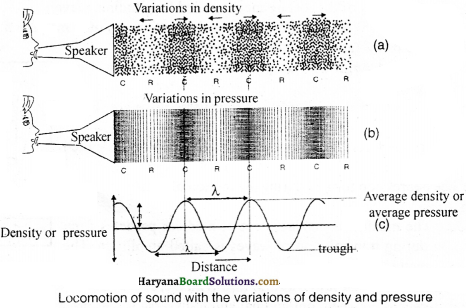On a certain time, both the density’ and pressure of the medium vary up and down from their average value. In fig. a region of high pressure is compression and low pressure is rarefaction. The peak is called crest and valley is called trough of wave.

Question 13.
What is the difference between the sound of violin and flute of an orchestra ?
Both the violin and flute travel in same medium and reach to our ear in same time, the speed of both is same, but both sounds are obtained from different characterstics. That is pitch. How the brain interprets the frequency of an emitted sound is called the pitch. Therefore, the sound of violin and flute are heard differently.Question 14.
Differentiate between low and high pitch through graph.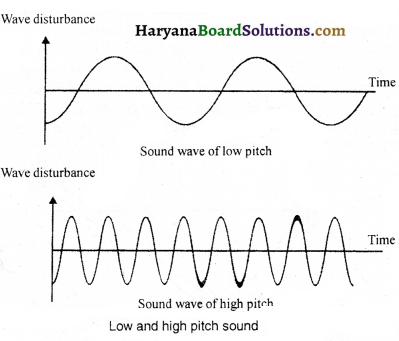Question 15.
What is the main difference between soft and loud sound ? Clarify it by graph.
The softness and loudness of sound depends upon the amplitude. For instance, knocking the door slowly produces a sound of’low amplitude and on loudly it produces a sound of high amplitude. Less energy is emerged in low amplitude and more energy is emerged in high amplitude. That is why the sound of low amplitude covers less distance while of high amplitude covers more distance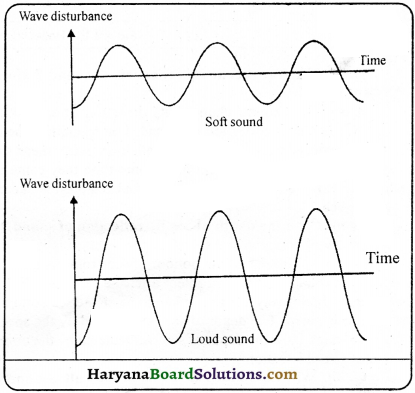Question 16.
Show by an experiment that speed of sound is more in liquids than in air.
Take a balloon full of water and rub its surface slowly. You will hear a sound. Now keep the balloon near your ear and rub it from other side, we will hear more and clear sound. It proves that the speed of sound is more in liquids than in air.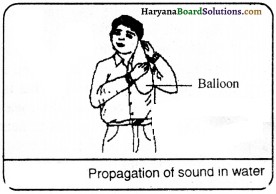Question 17.
How can we hear the sound of train coming from very far when ear is kept on track ?
We know that the speed of sound in iron on normal temperature is 5950 m/s. Therefore, we can hear the sound of train when it is so far by keeping the ear on line which is made of iron girders.

Question 18.
Describe an experiment to show the reflection of sound.
Take two identical wide tubes of plastic or card. Also take one sheet of plywood or metal. Arrange these according to fig. Keep a clock near the end of one of the tubes. Keep your ear near the other end of tube. Put a thick membrane between two tubes so that sound of watch cannot reach to your ears. Arrange the angle of second tube in such a way that you should be able to hear the sound of clock. You will see that in this situation both the tubes make equal angle to normal. This proves that sound is reflected.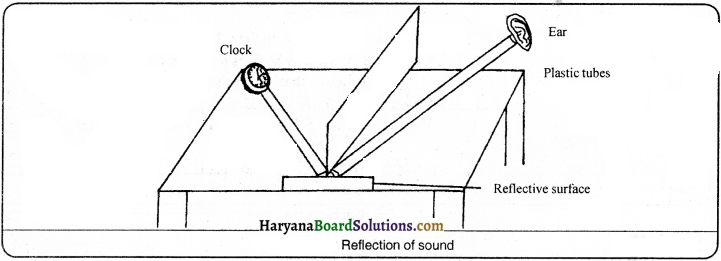Question 19.
What is meant by reflection of sound ? How is reflection of sound used to measure the depth of sea ?
When sound changes its direction after striking a surface, it is called reflection of sound. For example, when the sound is produced near the mouth of well, the wave of mean sound produced enter in the well and after some time they came back after reflecting from the surface of water and heard again like mean sound as echo.

To measure the depth of sea by reflection of sound:
The depth of sea is measured by sonar device. From this instrument, the sound signal is sent to the bottom of sea from the ship. This signal comes back to the instrument after the reflection from the sea bottom. In this way, the time taken to reach the sea bottom and reflection is noted. The depth of sea is measured by the speed of sound in water. Now the depth of sea is calculated by the time and speed of sound in water.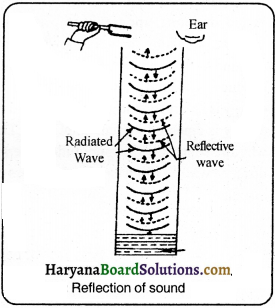Question 20.
What is the difference between ultrasound and echo ?
Ultrasound:
The frequencies more than 20 kHz are called ultrasonic sound. We cannot hear this sound. Some animals like dogs have the capacity to hear ultrasound. The bats have the capacity to produce and listen ultrasound.

Echo:
When sound is produced in deep well, big buildings, mountains, etc., the same sound is heard as mean sound after some time. This is known as echo. We can hear echo only when there is a minimum difference of 1/10 s between the mean sound and reflected sound. The minimum distance covered by the sound should be 34.4 metres to hear the echo. Thus, the object should be at a distance of 17.2 metres or more from the source of sound because the speed of sound at 22°C is 344 m/s. Time taken by sound to reach the obstacle and returning back should be 1/10 seconds. The sensation of sound in our brain lasts for 1/10 seconds.Question 21.
What is the audible range of different animals ?
The audible range of different animals is different. For example,
(1) Man – 20 Hz to 20,000 Hz.
(2) Dog and babies less than 5 yrs – upto 25 kHz.
(3) Rhino – 5 Hz.
(4) Whale and elephant – Infra sound.
(5) Dolphins, bats and porpoises – sounds of frequency more than 20 kHz.
(6) Moths – The moths can hear the high frequency squeaks of the bat.

Question 22.
What is the reason that we cannot hear the waves produced by earthquake whiie bats or dogs can?
Our ear can hear the waves of frequencies from 20 Hz to 20 kHz. This range of frequency is known as audible range. The frequency of the waves produced in earthquake does not lie in this limit. Therefore, we cannot hear these waves, but the bats and dogs are sensitive to these waves and can hear these waves.

Question 23.
Define infrasound waves, audible range and ultrasound.
1. Infrasound: Those waves whose frequency is less than 20 Hz are known as infrasound waves. We cannot hear them. i.e. earthquake wave.
2. Audible range : The waves of frequency between 20 Hz and 20 kHz is called audible range.
3. Ultrasound waves : Those waves whose frequency is more than 20 kHz is known as ultrasound waves. We cannot hear them also. Dogs and bats can hear these waves.

Question 24.
Write the uses of ultrasound.
The uses of ultrasound are following :
(1) Radar works on this principle.
(2) The milk exposed to them does not get spoilt for long.
(3) Sprouted is more in grain when affected by it.
(4) The cracks can be found in metal sheets melted by it.
(5) The bat find its prey by using this sound.
(6) The depth of sea can be measured by using it.
(7) It is used to wash clothes.
(8) It is used to find the cancer in body parts.
(9) It is used to cut hard substances and to join aluminium.Question 25.
A person has been kept in a closed room whose doors and windows are made of glass. When he makes a sound in the room, nobody hear that; but when he knocks the window, the outsider persons hear the knocking. Why ?
When a person in a room makes a sound, the waves produced by him is reflected from the glass. This produces weak vibrations in the glass, which is incapable to vibrate the air outside the room. As a result of it sound is not heard outside. Opposite to it, when the glass of window is knocked, the waves produced, reach the outer part of the glass and vibrate the air in its contact. Therefore, the sound of knocking is heard by persons.

Question 26.
What is sonic boom ? How is it harmful ?
Jet aircrafts produce shock waves in air. These shocking waves travel with supersonic speed. These waves carry a large amount of energy. The air pressure variation associated with this type of shock waves produces a very sharp and loud sound called the “sonic boom”. The shock waves produced by a supersonic aircraft have enough energy to shatter glass and even damage buildings. Sometime sonic boom produces such a loud noise which is beyond our intolerable limits. This can create trouble in our ears. Remember it, when supersonic aircraft flies, it continuously produces sonic boom around it.

Question 27.
Write a short note on whispering galleries.
The whispering gallery of dome of St. Paul’s Cathedral in London is very famous and serves an interesting example of reflection of sound. The gallery is running round the base in the inside of the dome in a circular form. When we whisper near any point of walls, the sound produced is reflected at every place. This can be clearly heard near the walls. But at some distance towards centre, let at point A, this sound can never be heard. One such gallery is in Gol Gumbaz at Bijapur in India.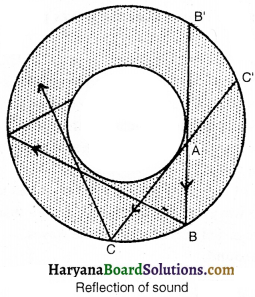Question 28.
What is SONAR ? What are its uses ?
SONAR: The instrument which produces sound waves and measures the small interval of time of reflected sound waves is known as SONAR.
Uses:
(1) To measure the depth of sea.
(2) To find the distance of groups of fishes.
(3) To locate the submarines and enemy torpedo.
(4) To detect cracks and flaws in metal blocks or sheets.Question 29.
How can the bat fly freely in dark by using the principle of sonar ?
Bats use the principle of SONAR naturally. Bat can produce ultrasound of frequency 120 kHz or 1.2 x 105 Hz and can hear them. The wavelength of such high frequencies is very less, approximately 0.29 cm. Bat is blind in reality, but in intense dark, it can fly without any difficult while crossing all the obstacles. It uses ultrasound to find its prey. When it flies, emits ultrasound signals of high frequency for small interval of time. These signals strike the surrounding objects and reflect. Thus, the bat calculates the time taken by ultrasonic waves after reflecting from any object. In this way the bat can fly freely in dark.

Question 30.
On what factors the speed of sound depends ?
The speed of sound depends on following factors:
1. Density of medium: The speed of sound is proportional to the density of medium. This is, the speed increases as the density increases and decreases with the decrease in density.
2. Temperature: Speed of sound increases with increase in temperature.
3. Wavelength: The speed of sound is proportional to wavelength.
4. State of medium: The speed of sound is maximum in solids, less in liquids and minimum in gases.

Question 31.
What is the audible range ? Write the name and uses of the frequencies more than this range.
Audible range:
A person can hear waves of frequency from 20 Hz to 20 kHz. This limit is called audible range.

The sound of frequency more than 20 kHz is called ultrasound. Its applications are as follows :
(2) It is used to find the cancer in body parts.Question 32.
How can we measure the depth of sea ?
An instrument is fitted on the ship to measure the depth of sea which sends ultrasound in sea level. These waves return back after reflecting from sea level and the receiver on ship receives these reflected rays. The time taken (t) by these ultrasonic waves to reach the sea level and return back and the speed of sound waves (v) help to calculate the distance (d) between the ship and sea level.
We know that,
Velocity =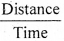or Distance = velocity x time.
= 2 d = v x t
d = $$\frac{\mathrm{v} \times \mathrm{t}}{2}$$
This will be the depth of sea at a certain place.

Essay Type Questions

Question 1.
What is sound ? Prove it by experiment that sound is produced by vibration.
Sound:
Sound is a form of energy which produces a sensation of hearing in our ears. It is produced by vibrating bodies. It can be proved by following experiments.

Experiment 1:
Take a tong. Hold it from the curved end and strike it against a surface or a stone. We will see the arms of the tong vibrating. Due to these vibrations a sound is heard. Repeat this process and bring the tong near to the ear. The sound of the tong is heard clearly.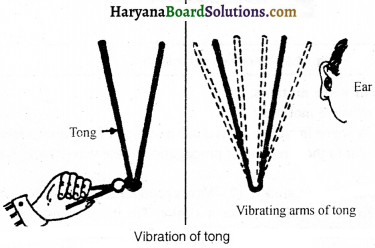Experiment 2:
Fix two nails on a wooden plank and tie a thin wire tightly between the two nails. Put a triangular piece of wood below the wire. Now pluck the wire and release it. A sound is heard. If we put a small piece ofpaper on the wire and again pluck it and release, the paper wil I fall dowm proving thatthe sound is produced due to vibrations.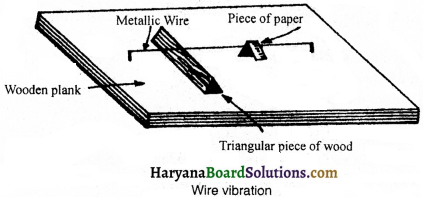Experiment 3.
Take a tuning fork. Strike it against a rubber pad, and make it stand straight on a table. A sound is produced which we can hear. Now bring a ball near to the prongs of the vibrating tuning fork. The prong strikes again and again with the ball. This proves the vibration of the tuning fork. If we bring our finger near to it, we can feel the vibrations. When we touch it the sound coming from the tuning fork will stop. This again proves that the sound is produced due to vibrations.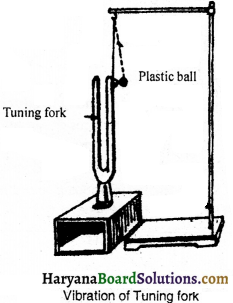Question 2.
Describe two types of wave motions.
There are two types of wave motions:
(a) Transverse waves:
A wave in which individual particles of medium vibrate about their mean position in a direction perpendicular to the direction of propagation of the wave through the medium, is called transverse wave.

Examples:
(1) Waves produced in a slinky
(2) Waves produced on the surface of the water.

(1) The waves produced in a slinky: Take a slinky, Tie its one end with a book to the wall. Hold its other end and give a jerk. The disturbance produced in the slinky moves from hand to the slinky and the particles of the slinky vibrate up and down from their mean position. This is an example of transverse wave.

(2) The wave produced on the surface of the water:
Throw a stone from a certain height in the still water of a pond then ripples are produced on the surface of the water which moves in all the directions. If we put a leaf on the ripples, the ripples will make the leaf move up and down at the same point. The raised upper part of the wave is called the ‘crest’ and the lower part is called the ‘trough’.(b) Longitudinal waves:
A wave in which the individual particles of medium vibrate back and forth from their mean position in direction parallel to the direction of propagation of the wave through the medium is called longitudinal wave.

Examples:
(1) Waves produced in a slinky
(2) Waves propagating in air.

(1) Waves produced in a slinky : Longitudinal waves can also be produced with slinky. Tie the one end of slinky with a hook on wall and stretch other end with your hand. Now press some turns in the small part of slinky between your fingers. The compression will reach the other end of slinky.

(2) The waves propagating in air : Take a tuning fork, suspend it forcefully with rubber pad. Its prongs will vibrate left-right. When one of its vibrating prongs moves in right, it press the air molecules due to this the air molecules compressed and the distance between them decreases. This is known as compression when the prong of vibrating fork moves in left, the vacuum is created in right side. To fill this, the air molecules expand to right side, and the distance among these air molecules increases which is known as rarefaction.

Question 3.
What are the characterstics of periodic waves ? Establish relationship among wave velocity, frequency and wavelength.
The periodic wave has following characterstics:
1. Amplitude: The magnitude of maximum displacement of vibrating particles from mean position is called amplitude of pendulum.
2. Time period: Time taken by a particle to complete one oscillation is called time period.
3. Frequency: The number of vibrations in a second by a vibrating particle is called frequency. It is denoted by Greek Letter (v). Its unit is Hertz (Hz).
4. Wavelength: The time in which the particles of medium complete one vibration, the distance covered by the wave in that period is called wavelength. It is denoted by X .
5. Velocity: The distance moved by the wave in unit time is called velocity of the wave. It is denoted by v.

The relation among frequency, wavelength and velocity of wave:
According to definition:

The distance covered by the wave T second = λ
The distance covered by the wave in 1 second = $$\frac {λ}{T}$$
But, the distance covered in 1 second = Velocity (v)
∴ v = $$\frac {λ}{T}$$ ………….(i)
If time taken in one vibration is T,
Then number of vibrations in T seconds = 1
Numbers of vibrations in 1 second = $$\frac {1}{T}$$
But, number of vibrations in 1 second is called frequency ‘υ’.
υ = $$\frac {1}{T}$$
Putting the value of y in equation (i)
v = $$\frac {λ}{T}$$ = λ $$\frac {1}{T}$$ [∴ $$\frac {1}{T}$$ = v]
y = λv
Thus, Velocity = wavelength x frequency.Question 4.
What is meant by reflection of sound ? Write three uses of it.
Reflection of Sound: When the sound strikes any surface it changes its direction which is called reflection of sound.
Uses of reflection of sound: The practical uses of reflection of sound are following :

1. Megaphones:
You have seen the use of megaphones to address the crowd in fairs or tourists places. Megaphone is simply a horn shaped instrument. When a person speaks in the megaphone, the sound waves are prevented from spreading out due to repeated and successive reflections from the walls of megaphone tube. For this reason, loudspeakers have horn shapes openings.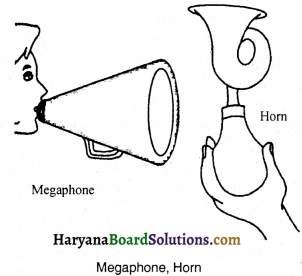2. Ear trumpet or Hearing aid:
People with hard of hearing may need a hearing aid. Hearing aid is an electronic battery operated device. The hearing aid receives sound through a microphone. The microphone converts the sound waves to electrical signals. These electrical signals are amplified by an amplifier. The amplifier electric signals are given to a speaker of the hearing aid. The speaker converts the amplified electrical signal to sound and sends to the ear for clear hearing.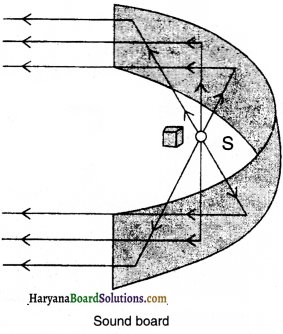3. Sound drum or boards : The reflection of sound is also possible from curved surfaces. This fact is used in bigger halls to spread the sound in whole hall. Sound drums are used for this purposes. The speaker S is focussed at S sound drum. Sometimes a curved sunboard may be placed behind the stage so that the sound after reflecting from the sound board, spreads evenly across the width of the hall as shown in fig.

Question 5.
Describe the different parts of human ear.
There are three main parts of human ear:
(a) External ear : It is that part which is seen from outside. It consists of projecting part known as pinna and the passage auditory canal which is connected to a thin membrane called the eardrum or thin panic membrane.
1. Pinna: It consists of cartilage and muscles. Its lower part is soft and elastic. Pinna collects the sound waves and gives direction to them. It is joined with auditory canal.2. Auditory Canal: It is a slant about 3-4 cm long. Its skin contains hair and micro glands and liquid comes out of them. This is called ear wax. It prevents the ears from dust particles and insects.

3. Eardrum: There is a thin membrane in the end of auditory canal which is called eardrum. It sends the sound waves to middle ear.

(b) Middle ear: The middle ear is like a air filled room. Middle ear is attached to throat through a canal which is called ‘eustachian canal’. This canal maintains the air pressure in two sides of eardrum. The middle ear contains three tiny bones –
(i) hammer
(ii) anvil
(iii) stirrup.

These three bone are together called ear ossicles.
(c) The Inner Ear: It contains
(i) cochlea
(ii) auditory nerves
(iii) semi-circular canals.

(i) Cochlea: The shape of cochlea is spiral and has two half turns. It contains a liquid which is called ‘andolimph’. It helps in hearing.
(ii) Auditory nerve: It is a small chamber which is connected with cochlea from front and from back with semi circular canals.
(iii) Semi-circular canals: These are three in number and arranged at right angles to-each other in three different planes. The ends of auditory canals swim in ‘andolimph.’

Important Table

 State Substance Speed in m/s Solids Aluminium 6420 Nickel 6040 Steel 5960 Iron 5950 Brass 4700 Glass (Flint) 3980 Liquids Water (sea) 1531 Water (distilled) 1498 Ethanol 1207 Methanol 1103 Gases Hydrogen 1284 Helium 965 Air 346 Oxygen 316 Sulphur dioxide 213

Numerical Questions

Question 1.
The frequency and wavelength of a sound wave is 2 kHz and 25 cm respectively. How much time will it take to move a distance of 1.5 km ?
Solution:
Here, Frequency v = 2 kHz = 2000 Hz
Wavelength λ = 25cm = 0.25 m
We know that, velocity of wave v= wavelength x frequency v = λv
v = 0.25m x 2000 Hz = 500m/s
Time taken by wave to move a distance of 1.5 km,
t = $$\frac{\mathrm{d}}{\mathrm{v}}=\frac{1.5 \times 1000 \mathrm{~m}}{500 \mathrm{~ms}^{-1}}=\frac{15}{5}$$s = 3s
Thus, sound will take 3 s to travel on distance of 1.5 km.

Question 2.
A person clapped his hands near a cliff and heard the echo after 6 s. What is the distance of the cliff from the person if the speed of the sound, v is taken as 346 ms-1 ?
Solution:
Given, Speed of sound, (v) = 346 ms-1
Time taken for hearing the echo, (t) = 6s
Distance travelled by the sound = v x t = 346 ms-1 x 6s = 2076 m
In 5 s sound has to travel twice the distance between the cliff and the person. Hence, the distance between the cliff and the person = 2076 m/2= 1038 m.Question 3.
A ship sends out ultrasound that returns from the seabed and is detected after 3.42 s. If the speed of ultrasound through seawater is 1531 m/s, what is the distance of the seabed from the ship ?
Solution:
Given, time between transmission and detection, (t) = 3.42 s.
Speed of ultrasound in sea water, (v) =1531 m/s
Distance travelled by the ultrasound = 2 x depth of the sea = 2d
Where d is the depth of the sea.
2 d = speed of sound x time = 1531 m/s x 3.42 s = 5236 m
d = 5236 m/s = 2618 m
Thus, the distance of the seabed from the ship is 2618 m or 2.62 km.

Question 4.
Two children are standing at two opposite ends of an iron pipe. One boy hit one end of pipe with stone. Find the ratio of the time taken by the sound to travel through iron and air (Given, speed of sound in air is 344 m/s and in iron 5130 m/s).
Solution:
Here,
Let length of the pipe = L m
Velocity of sound in air (v1) = 344 m/s
Velocity of sound in iron (v2) = 5130 m/s
∴ Time taken by sound in the air (T1) = $$\frac{\mathrm{L}}{\mathrm{v}_1}=\frac{\mathrm{L}}{344}$$s
Time taken by sound in iron (T1) = $$\frac{\mathrm{L}}{\mathrm{v}_2}=\frac{\mathrm{L}}{5130^{\mathrm{s}}}$$
The ratio between two time = $$\frac{T_1}{T_2}=\frac{\frac{\mathrm{L}}{344}}{\frac{\mathrm{L}}{5130}}$$
= $$\frac{\mathrm{L}}{344} \times \frac{5130}{\mathrm{~L}}$$
= $$\frac{14.9}{1}$$
T1 : T2 = 14.9 : 1

Question 5.
A boat at anchor is rocked by waves whose crests are 100 m apart and whose velocity is 20 m/s. How often do the crest reach the boat?
Solution:
Here,
The distance between two consecutive crests of wave = 100 m
Velocity of wave (v) = 20 m/s
Frequency of wave (v) = $$\frac{v}{\lambda}=\frac{20}{100}$$ Hz = 0.2 HzQuestion 6.
A source of wave produces 40 crests and 40 troughs in 0.4 s. Find the frequency of the wave.
Solution:
Here,
Number of wave crests = Number of wave troughs = 40
time = 0.4 s
Number of crests per second Number of troughs per second =$$\frac{40}{0.4}$$ = 100
Thus, the frequency of wave = 100 Hz

Question 7.
Using the SONAR, sound pulses are emitted at the surface. The pulses after bdng reflected from the bottom are detected. If the time interval from the emission to the detection of the sound pulse is 2s, find the depth of the wster. IVelocity of sound ¡n water is 1498 rn/sI
Solution:
The velocity of sound in water (y) = 1498 m/s
Time taken in coming and going pulse = 2 s
Thus, time taken in going pulse = $$\frac{2}{2}$$ = 1 s
Depth of water = velocity x time = 1498 x 1 = 1498 metres

Question 8.
A child hears an echo from a cliff 4 seconds after the sound from a powerful cracker is produced. How far away is the cliff from the child?
Solution:
Total time taken by sound in going and returning back to the child = 4 s
The time taken by sound in going to the child = $$\frac{4}{2}$$ = 2s
The velocity of sound in air 344 = m/s
Thus, the distance between the child and the cliff = velocity x time = 344 x 2 = 688 m

Question 9.
A stone is dropped into a well 44.1 metres deep. The sound of the splash is heard 3.13 s after the stone is dropped. Find the velocity of sound in air.
Solution:
Here,
Depth of the well (s) = 44.1 m
Suppose time taken by the stone to reach the well = t1 s
And the time taken by the sound to reach on water = t2 s
According to the question t1 + t2 = 3.13
second u = 0, g =9.8 m/s2

We know that s = u t + $$\frac{1}{2}$$ gt2
44.1 = 0 x t1 + gt12 (For t1)
or 441 = $$\frac{1}{2}$$ x 9.8 x t12
or t12 = $$\frac{44.1 \times 2}{9.8}$$ = 9
or t1 = 3s
t2 = 3.13 – t1 = 3.13 – 3 = 0.13 s
Velocity of sound == $$\frac{44.1}{0.13}$$ = $$\frac{441 \times 100}{10 \times 13}$$ = 339.2 m/s

Question 10.
A tuning fork produces waves of frequency 512 Hz by vibration, which moves with a velocity of 330 m/s. Find its wavelength.
Solution:
Here,
Frequency (υ) = 512 Hz
Wave velocity (v) = 330 mis
Wavelength (λ) = ?
We know that,
v = υ λ
Or λ = $$\frac{v}{v}=\frac{330}{512}$$ m = 0.645 m

Question 11.
What is the speed of that water wave whose wavelength ¡s 20cm and frequency is 20 Hz.
Solution:
Here,
Wavelength (λ) = 20 cm = $$\frac{20}{100}$$ m = 0.2m
Frequency (υ) = 20 Hz
Velocity of wave (v) = ?
We know that, v = υ λ = 20 x 0.2 = 4 m/sQuestion 12.
Find the frequency of that wave whose time period is 0.02 s.
Solution:
Here,
Time period (T) = 0.02 s
Frequency (υ) = ?
We know that
υ = $$\frac{\mathrm{I}}{\mathrm{T}}=\frac{1}{0.02}$$ = $$\frac{100}{2}$$ = 50 Hz

Question 13.
If the frequency of a tuning fork is 400 Hz and the speed of sound in air is 320 m/s, find the distance covered by the sound when tuning fork completes 20 vibrations.
Solution:
Here,
Wave velocity (v) = 320 mIs
Frequency (υ) = 400 Hz
We know that v = υ λ
or λ = $$\frac{v}{v}=\frac{320}{400}$$ = 0.8 m
Thus, the distance covered in I vibration = 0.8 m
The distance covered in 20 vibrations = 20 x 0.8 = 16 m

Question 14.
An object completes 6000 vibrations in one minute. If the speed of sound in air is 330 m/s, find the (a) frequency of vibrations (b) wavelength of produced waves.
Solution:
(a) 1 minute = Number of vibrations in 60 s = 6000
Number of vibrations in I second = $$\frac{6000}{60}$$ = 100
Thus, frequency (υ) = 100 Hz

(b) Here, velocity of wave (v) = 330m1s
Frequency (υ) loo Hz
Wavelength (λ) = ?
We know that v = υλ
or λ = $$\frac{\mathrm{v}}{v}=\frac{330}{100}$$ = 3.3 m

Question 15.
The velocity of light wave is 3 x 105 km/s and wavelength is 6 x 107 m. Find its frequency.
Solution:
Here,
Velocity of wave (v) = 3 x 105 km/s
3 x 105 x 1000.m/s
3 x 108 m/s
Wavelength (λ) = 6 x 10-7 m
Frequency (υ) = ?
We know that v = υλ
υ = $$\frac{v}{\lambda}=\frac{3 \times 10^8}{6 \times 10^{-7}}$$ = 0.5 x 1015 Hz
Thus, frequency is 0.5 x 1015 Hz.Question 16.
An observer is standing at a distance of 200 metres from a cliff and whistles. If the speed of sound is 332 mIs, when the echo of whistle will be heard?
Solution:
Distance of observer from cliff = 200 m
To hear the echo,
Total distance covered = 200 + 200 400 m
Speed of sound = 332 m/s
Time =$$\frac{400}{332}$$ = 12 s

Question 17.
A Sound signal is send from a boat. That signal return after 0.8s by reflecting from the sea bottom. Find the velocity of sound in water if the depth of sea is 600m.
Solution:
Here,
Depth of sea = 600 m
Distance travelled by the signal = 600 + 600 = 1200 m
Time = 0.8s
Velocity of Sound in water == $$\frac{1200}{0.8}$$ m/s
1500 m/s

Question 18.
A sound signal is send from a boat. That signal return after 0.8 s by reflecting from the sea bottom. If the velocity of sound is 1500 mIs, then find the depth of sea.
Solution:
Here,
Velocity of sound in water = 1500 mis
Time taken = 0.8 s
Distance travelled by sound = Velocity of sound x time
1500 x 0.8 = 1200m
Thus Depth of sea latex]\frac{1200}{2}[/latex] = 600 m

Practical Work

Experiment 1.
To study the vibrations produced by tuning fork.

Procedure:
(i) Suspend a small plastic ball by a thread from a support.
(ii) Bring the non-vibrating tuning fork near the ball and observe the situation.
(iii) Now bring the vibrating tuning fork near the ball and observe the situation.
In first situation, the ball does not move far from tuning fork while in second situation, the ball moves far as the tuning fork is taken near to it, because the prong of tuning fork applies the force on ball and pushes it backward.Experiment 2.
To study the vibrating parts of musical instruments.

Procedure:
Go into the music room of your school and know about the system of producing sound in musical instruments from music teacher.

For example:
(i) In drum: by the vibration of membrane.
(ii) Sitar: by vibration in wire.
(iii) Ektara: by vibration in wire.
(iv) In flute: by air column.
(v) In water wave: by water.Experiment 3.
To produce longitudinal wave by slinky.

Procedure:
(i) Take a slinky. Ask your friend to hold one end and you hold the other end.
(ii) Give it a sharp push towards your friend. A special motion will be seen in slinky while repeated.
Mark a dot on the slinky and repeat the process again. The dot on the slinky will move back and forth parallel to the direction of the propagation of the disturbance. This is longitudinal wave.

Experiment 4.
To understand the reflection of sound.

Procedure:
Take a hallow cylinder of cardboard opened from both ends. Keep a small clock near the open end of cylinder and keep the wooden block in such a way that the person standing on the other end of the cylinder can hear the sound of tik-tik of clock. Now keep the other wooden block far from the other open end of cylinder. At this time sound reaches the ears after striking from surface. The change in the direction of sound after striking from any surface is called reflection of sound.

Quick Review of the Chapter

1. The sound of a bell is
(A) mechanical energy
(B) sound energy
(C) heat energy
(D) light energy
(B) sound energy

2. Sound is produced due to
(A) striking
(B) vibration
(C) beating
(D) rubbing
(B) vibration

3. Sound does not travel/transmit in:
(A) solids
(B) liquids
(C) gases
(D) vacuum
(D) vacuum

4. Vave is a …………
(A) disturbance
(B) compression
(C) rarefaction
(D) vibration
(A) disturbance

5. A region of high pressure is called :
(A) wave
(B) compression
(C) rarefaction
(D) disturbance
(B) compression6. In which of the following the speed of sound maximum?
(A) Dry air
(B) steel
(C) wet air
(D) water
(B) steel

7. For sound propagation, which is the most common medium?
(A) water
(B) earth
(C) air
(D) space
(C) air

8. Which are waves produced in water?
(A) longitudinal
(B) transverse
(C) partially both (A) and (B)
(D) sometime (A) and sometime (B)
(B) transverse

9. The SI unit of wave velocity is:
(A) m
(B) mis
(C) km
(D) kmls
(B) m/s

10. Which of the following is represented by λ (lambda)?
(A) Wavelength
(B) Wave velocity
(C) Frequency
(D) Amplitude
(A) Wavelength

11. The transfer of takes place in sound propogation.
(A) mass
(B) both (A) and (C)
(C) energy
(D) none of these
(C) energy12. The longitudinal waves are produced in :
(A) solids
(B) liquids
(C) gases
(D) all three
(D) all three

13. The transverse waves are produced ¡n :
(A) solid and liquids
(B) liquids and gases
(C) gases and solids
(D) all three
(A) solid and liquids

14. Light is wave.
(A) transverse
(B) mechanical
(C) longitudinal
(D) electromagnetic
(A) transverse

15. The frequency of a source of sound is loo Hz. How many times does it vibrate in Half an hour?
(A) 1,80,000
(B) 90,000
(C) 60,000 (D) I ,00,000
(A) 1,80,000

16. The distance between is called wavelength.
(A) two consecutive compressions
(B) two consecutive rarefactions
(C) a compression and a rarefaction
(D) both (A) and (B)
(D) both (A) and (B)

17. The SI unit of wavelength is.
(A) metre
(B) joule
(C) erg
(D) newton
(A) metre

18. What is the unit of frequency of a sound wave?
(A) Hertz
(B) Metre
(C) erg
(D) Joule
(A) Hertz19. The SI unit of time period is:
(A) Hertz
(B) Metre
(C) Second
(D) Nm
(C) Second

20. The magnitude of the maximum distrubance in the medium on either side of the mean is called
(A) frequency
(B) amplitude
(C) velocity
(D) none of the above
(B) amplitude

21. What is represented by AR?
(A) frequency
(B) velocity
(C) amplitude
(D) time period
(C) amplitude

22. The sound of single frequency is called
(A) note
(B) tone
(C) noise
(D) none of these
(B) tone

23. A sound which is produced due to mixture of several frequencies is called
(A) note
(B) tone
(C) noise
(D) lyrics
(A) note

24. The amount of sound passing each second through unit area is called . of sound.
(A) loudness
(B) intensity
(C) pitch
(D) frequency
(B) intensity

25. In which medium the speed of sound is maximum at 25°C?
(A) glass
(B) steel
(C) aluminium
(D) nickel
(C) aluminium26. In which liquid the speed of sound is maximum at 25°C?
(A) sea water
(B) distilled water
(C) ethanol
(D) methanol
(A) sea water

27. To listen the echo clearly the minimum distance of reflector should be:
(A) 172m
(B) 17.2m
(C) 344 m
(D) 344 m
(B) 17.2 m

28. In which gas the speed of sound is maximum at 25°C?
(A) oxygen
(B) air
(C) hydrogen
(D) helium
(C) hydrogen

29. The sensation of sound can remain about second in our brain.
(A) 0.1
(B) 0.2
(C) 0.3
(D) 0.4
(A) 0.1

30. Sound obsorber substances are installed walls and roofs of building to reduce
(A) echo
(B) sound reflection
(C) reverberation
(D) supersonic sound
(C) reverberation31. The principle of reflection of sound is used:
(A) in megaphone and horns
(B) stethoscope
(C) in concert hail, cinema halls
(D) in all aboe mentioned
(D) in all above mentioned

32. What is hearing range of sound in man?
(A) 10-15 Hz
(B) 20-20.000Hz
(C) 30.000 Hz-40,000 Hz
(D) 45,000 Hz-50,000 Hz
(B) 20-20,000 Hz

33. How much Hz sound can the children and dogs of age below S hear?
(A) to25kHz
(B) to30kHz
(C) to 35 k Hz
(D) to 45 k Hz
(A) to 25 k Hz

34. Sounds of frequencies below are called infrasonic sound.
(A) 20 Hz
(B) 200 Hz
(C) 2000 Hz
(D) 20000 Hz
(A) 20 Hz

35. Frequencies higher than is called ultrasonic sound.
(A) 10kHz
(B) 20kHz
(C) 25 k Hz
(D) 30 k Hz
(B) 20 k Hz

36. Ultrasonic sound is used :
(A) for cleaning dust, oilness and dirt
(B) to detect cracks in bodies of metal
(C) in ECG and ultrasound
(D) in all above mentioned
(D) in all above mentioned

37. SONAR is used to measure the of bodies under sea water.
(A) distance
(B) direction
(C) speed
(D) all above mentioned
(D) all above mentioned

38. Which part is lamella of an ear?
(A) outer part
(B) midd’e ear
(C) inner ear
(D) auditory nerve
(A) outer part39. How are the waetength and frequency of a sound wave related to its speed ?
(A) λ= vυ
(B) v = $$\frac {υ}{λ}$$
(C) υ = λ υ2
(D) v = υλ